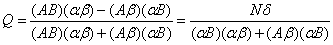# Statistics Assignment Help With Yule’s coefficient of association

## 9.5.1 Yule's coefficient of association:

As a measure of the intensity of association between two attributes A and B, G. Udny Yule gave the coefficient of association Q, defined as follows:If A and B are independent, δ= 0 à Q= 0.If A and B are completely associated, then

Either (AB) = (A) à (Aβ) = 0

Or (AB) =(B) à (αB) = 0

And in each case Q = +1.

If A and B are in complete dissociation then either (AB) = 0 or (αβ) = 0 and we get Q = -1.

Hence, -1 ≤ Q ≤ 1